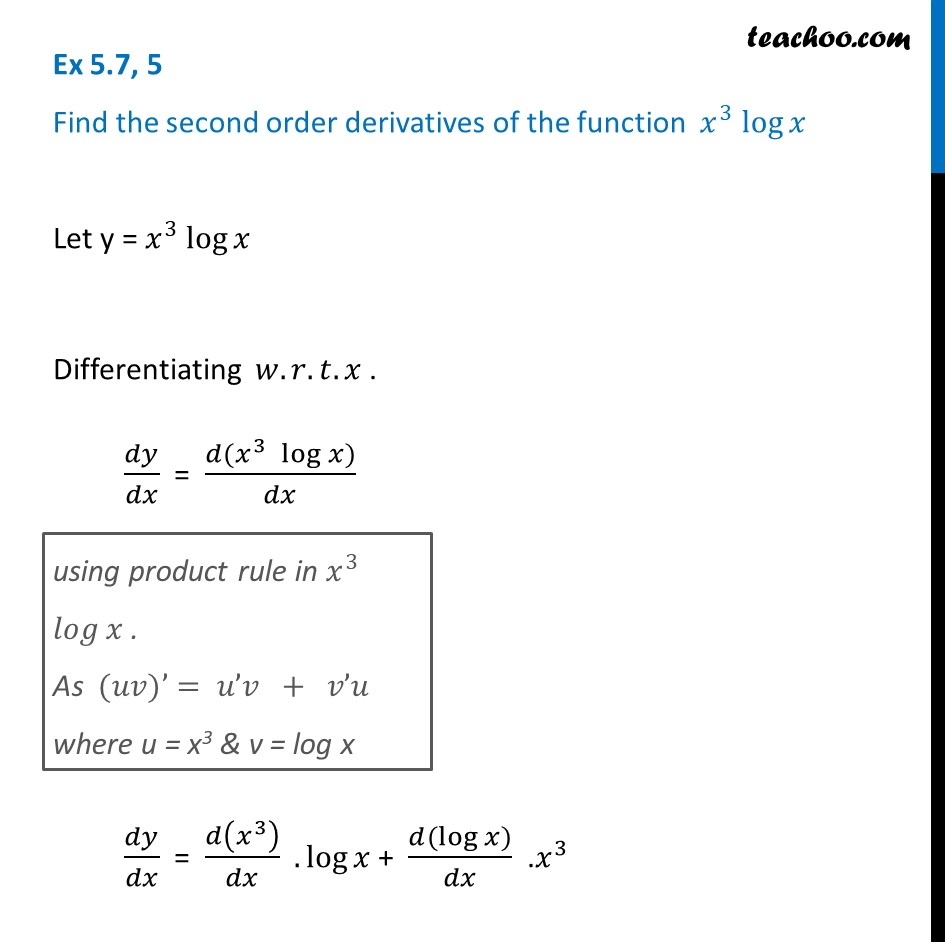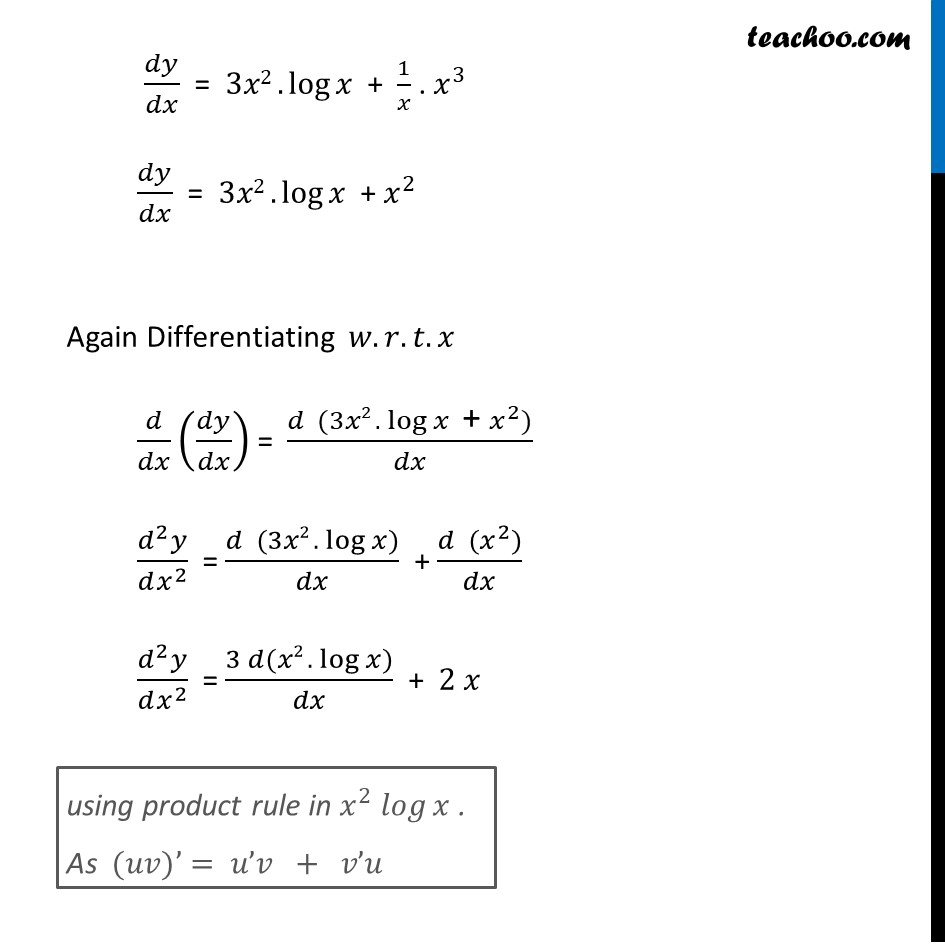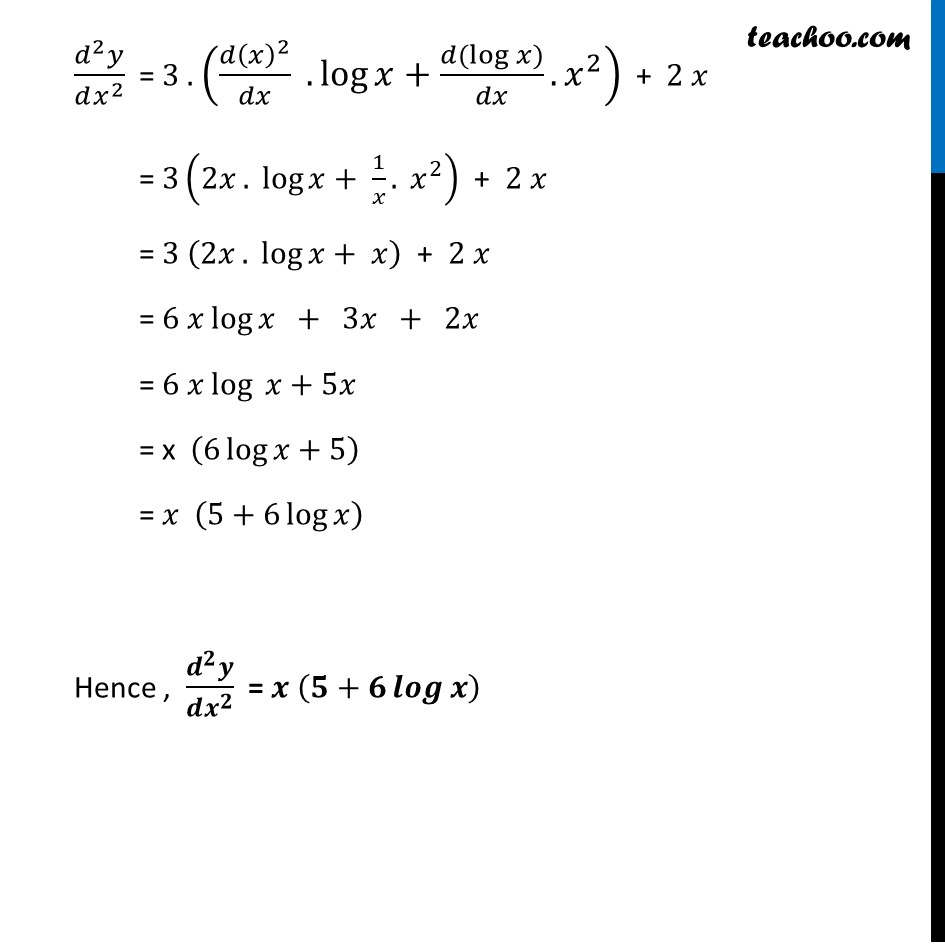Ex 5.7

Chapter 5 Class 12 Continuity and Differentiability
Serial order wiseLearn in your speed, with individual attention - Teachoo Maths 1-on-1 Class

### Transcript

Ex 5.7, 5 Find the second order derivatives of the function 𝑥^3 log⁡𝑥 Let y = 𝑥^3 log⁡𝑥 Differentiating 𝑤.𝑟.𝑡.𝑥 . 𝑑𝑦/𝑑𝑥 = (𝑑(𝑥^3 " " log⁡𝑥))/𝑑𝑥 𝑑𝑦/𝑑𝑥 = 𝑑(𝑥^3 )/𝑑𝑥 .log⁡𝑥 + (𝑑(log⁡𝑥))/𝑑𝑥 .𝑥^3 using product rule in 𝑥^3 𝑙𝑜𝑔⁡𝑥 . As (𝑢𝑣)’= 𝑢’𝑣 + 𝑣’𝑢 where u = x3 & v = log x 𝑑𝑦/𝑑𝑥 = 3𝑥2 . log⁡𝑥 + 1/𝑥 . 𝑥^3 𝑑𝑦/𝑑𝑥 = 3𝑥2 . log⁡𝑥 + 𝑥^2 Again Differentiating 𝑤.𝑟.𝑡.𝑥 𝑑/𝑑𝑥 (𝑑𝑦/𝑑𝑥) = (𝑑 (3𝑥2 . log⁡𝑥 "+ " 𝑥^2))/𝑑𝑥 (𝑑^2 𝑦)/(𝑑𝑥^2 ) = (𝑑 (3𝑥2 . log⁡𝑥))/𝑑𝑥 + (𝑑 (𝑥^2))/𝑑𝑥 (𝑑^2 𝑦)/(𝑑𝑥^2 ) = (3 𝑑(𝑥2 . log⁡𝑥))/𝑑𝑥 + 2 𝑥 using product rule in 𝑥^2 𝑙𝑜𝑔⁡𝑥 . As (𝑢𝑣)’= 𝑢’𝑣 + 𝑣’𝑢 (𝑑^2 𝑦)/(𝑑𝑥^2 ) = 3 . ((𝑑(𝑥)^2)/𝑑𝑥 .log⁡〖𝑥+(𝑑(log⁡〖𝑥)〗)/𝑑𝑥〗. 𝑥^2 ) + 2 𝑥 = 3(2𝑥 . log⁡〖𝑥+ 1/𝑥〗. 𝑥^2 ) + 2 𝑥 = 3 (2𝑥 . log⁡𝑥+ 𝑥) + 2 𝑥 = 6 𝑥 log⁡𝑥 + 3𝑥 + 2𝑥 = 6 𝑥 log ⁡𝑥+5𝑥 = x (6 log⁡〖𝑥+5〗 ) = 𝑥 (5+6 log⁡𝑥 ) Hence , (𝒅^𝟐 𝒚)/(𝒅𝒙^𝟐 ) = 𝒙 (𝟓+𝟔 𝒍𝒐𝒈⁡𝒙 )